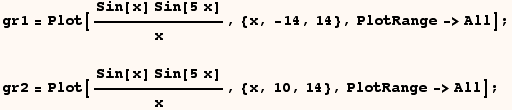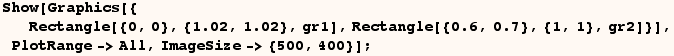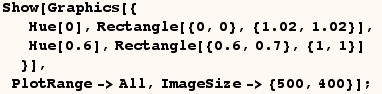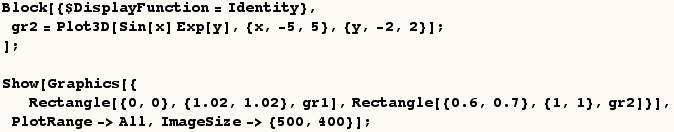Rectangle

Rectangle is a griphics primative. The Rectangle coordinates can be Scaled, Offset, or (Scaled and Offset) and these features are explained in another section.  Except for non-stnadard coordinates the basic use of Rectangle is well documented.  On the other hand  Rectangle has an interesting feature in that it can take a graphics object as a third argument, and I demonstrate this feature here.

Consider the two plots made with the next cell.A nice presentation of these plots would have the graphic with a smaller plot range in the upper right corner used to display the one with a larger plot range.  We can do that by giving Rectangle these graphics as third arguments in the next cell.To explain what happens above consider the next cell which is similar to the previous cell.  The next cell makes a red rectangle and a blue in the upper left corner of the red rectangle (Hue is red, and Hue[0.6] is blue). In the previous cell the first Rectangle had (gr1) as a third argument, and that makes the rectangle filled in with graphic (gr1)  instead of a solid color.  Then the second Rectangle in the previous cell had (gr2) as a third argument, and that makes the second rectangle filled in with graphic (gr2) instead of a solid color.You can further dress up a graphic with additional graphics primatives as I do in the next cell.The third argument given to Rectangle doesn't need to have the head Graphics.  Any type of Mathematica graphics can be given.  In the next cell one of the rectangles is given Graphics3D as a third argument.Created by Mathematica  (May 16, 2004)

Back to Ted’s Tricks index page